Courses

# Worksheet Questions- Rational Numbers Class 8 Notes | EduRev

## Mathematics (Maths) Class 8

Created by: Full Circle

## Class 8 : Worksheet Questions- Rational Numbers Class 8 Notes | EduRev

The document Worksheet Questions- Rational Numbers Class 8 Notes | EduRev is a part of the Class 8 Course Mathematics (Maths) Class 8.
All you need of Class 8 at this link: Class 8

1. Fill in the blanks:

(i) The product of a number and its product is ________.

ii) The rational number ________ has no reciprocal.

(iii) The reciprocal of the reciprocal of a number is the ________.

(iv) The rational number ________ is neither positive nor negative.

(v) ________ is the only rational number which is equal its additive inverse.

2. Write:

(i) A rational number which has no reciprocal.

(ii) A rational number whose product with a given rational number is equal to the given rational number.

(iii) A rational number which is equal to its reciprocal.

3. Verify that: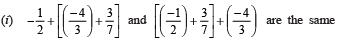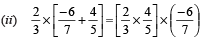4. Find: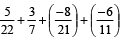5. Find: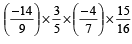6. Write the additive inverse of:

(i) 7/19

(ii) -21/112

7. Verify that –(–p) = p for p = -11/31

8. Find: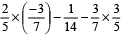9. Write the reciprocal of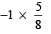1. (i) 1
(ii) 0
(iii) the number itself
(iv) 0
(v) 0

2. (i) 0
(ii) 1
(iii) 1

4. -125/462

5. 1/2

6. (i) -7/19
(ii) 21/112

8.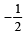9.## Mathematics (Maths) Class 8

217 videos|147 docs|48 tests

,

,

,

,

,

,

,

,

,

,

,

,

,

,

,

,

,

,

,

,

,

;